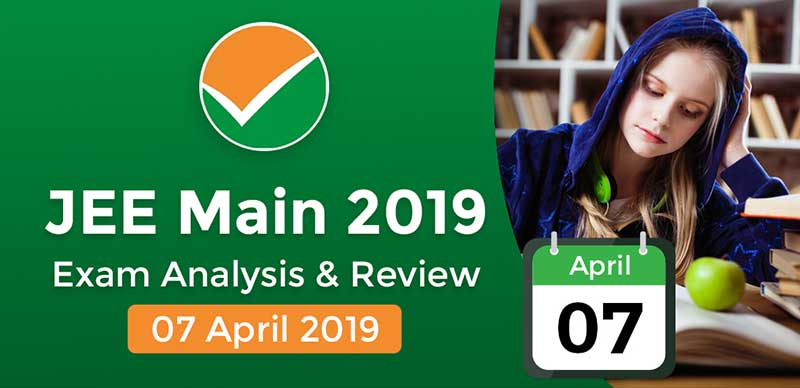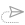### JEE Main 2019: Exam Analysis For Paper 2 (Shift 1)

Shift 1 of JEE Main 2019 Paper 2, on April 7, has ended.

You can now check JEE Main 2019 Exam Analysis by students and experts from this page.

Paper 2 was easier in comparison to Jan exam. Know which was the easiest and most difficult section in the exam.

The questions for drawing test are also out now. Know details about JEE Main 2019.

As per the candidates, the aptitude section of the paper was the easiest of all. Overall the paper was moderate in both the shifts. Mathematics was found to be moderate to difficult.

The drawing section of JEE Main Paper 2 was the most time taking as per the candidates.

JEE Main Paper 2 is of 390 marks and candidates need to solve 30 question of Maths, 50 MCQ for Aptitude and 2 Drawing questions.

The question paper of JEE Main 2019 paper 2 has the following 3 sections. And thus, in this part, the section-wise analysis of the paper is made available after the exam is over:

Part 1 – Mathematics

For the April attempt, candidates have claimed that the questions in this attempt were easier. There were 4 questions from Integration in the mathematics section. There were Questions from Calculus and 3-D were tricky. Maths although was difficult for most, some student found it easy to moderate. There were tricky questions from class 11. The division of the questions was 75% and 25% ratio from class 12 and 11 respectively. There were no questions that were repeated from the January session of JEE Main 2019.

Some of the questions asked in the paper are given below for candidates:

• If OP is a line of slope 1/√3 joining origin and point P on the parabola y² = 3x. A normal is drawn is P which cuts the axis of the parabola at Q. Then the distance QS is (Where S is the focus of the parabola?
a) 45/4
b)39/4
c)35/7
d) none of these
• In an increasing GP if the sum of the first and sixth term is 66 and the product of the second and fifth term is 128, then the sum of the first six terms of this GP is?
• Complete the following series: 38, 190, 950,…..?

Ans: 4750

•  If f (x) = a log |x| + bx³ + cx² and -1 and 1 are the point of inflection, then?

Ans: f”(1) + f”(-1) = 0

Part 2- General Aptitude

The aptitude section was easy enough to be solved in just 20 to 25 minutes. There were no tricky questions as such.

Some of the questions asked in the paper are given below for candidates:

• Which is the Fort of Hyderabad

Ans: Golconda Fort

• Where is Sas-bahu temple??

• Where is leaning tower of Pisa?

Ans: Piazza del Duomo, 56126 Pisa PI, Italy

•  Which is the highest tower in Bengaluru?

Ans: Mantri DSK Pinnacle

• How does an escalator move??

Ans: A typical escalator uses a 100 horsepower motor to rotate the gear

•  Where was Zaha Hadid born?

Part 3 – Drawing skills

Drawing sections was one of the most time taking sections. The sketching took most of their time. The questions were based on imagination skills and also to judge the understanding skills of the candidates.

Some of the questions asked in the paper are given below for candidates:

• Design a square table cloth.
• Draw a classroom scene in which the professor and student can be seen from behind.
• Students had to make an aesthetic image out of a cube using their imagination.
• Students have to replicate an image of the flower, as accurately as they can.

Student Reaction:

• There were no questions repeated from the January exam paper.
• Few students have said the Probability was a bit difficult.
• Drawing section was difficult for Shift 1
• Questions related to Calculus and 3-D from Maths section were tricky.
• There were 04 questions were from Integration of Mathematics section.
• Mathematics was moderate-difficult
• Some students said that questions from the Aptitude section were easy to solve. However, a few questions were out of syllabus.
• The exam was overall Moderate.
• Some students have commented that the paper was easy in comparison to the January attempt.16 October, 2021, 10:17 am##### JEE Advanced 2021 Result On This Date, Check Admission Process Here

11 October, 2021, 11:11 am##### JEE Main Paper 2 Result 2021 Declared; Direct Link Available Here

6 October, 2021, 10:46 am##### JEE Advanced 2021 : response sheet released, Full Details Inside

6 October, 2021, 10:22 am##### JEE Advanced Exam Analysis 2021 : Section Wise Memory Based Questions of Paper I & II Held On 03/10/2021

4 October, 2021, 10:15 am27 September, 2021, 10:51 amWe are providing something unique, useful and most importantly fun. By giving students a tool to find instant solutions to their doubts, we’re trying to make every student self-sufficient in practicing & completing their homework×